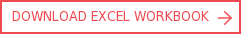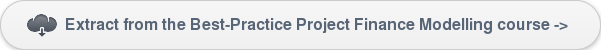View More Content
SHARE

# LLCR - Loan life coverage ratioCategoriesTutorialsThe loan life coverage ratio or loan life cover ratio (LLCR) is one of the most commonly used debt metrics in project finance and also often used in corporate debts. Unlike period-on-period measures such as the debt service cover ratio (DSCR), the LLCR provides the lenders with a measure of the number of times the project cash flow over the scheduled life of the loan can repay the outstanding debt balance.

This tutorial looks at the LLCR calculation, common errors and oversights made when modelling LLCR, and a method to check the calculation.### KEY LEARNINGS

• Definition of LLCR

• LLCR calculation

• Common errors an oversights made when modelling the LLCR

• Checking the calculation of LLCR

## Interpretation of the loan life cover ratio (LLCR) - example 1

An LLCR of 2.00x means that the cashflow available for debt service (CFADS), on a discounted basis, is double the amount of the outstanding debt balance.

## Interpretation of the loan life cover ratio (LLCR) - example 2

An LLCR of 1.00x means that the CFADS, on a discounted basis, is exactly equal to the amount of the outstanding debt balance. The movement of a key variable to achieve an LLCR of 1.00x is an important measure of the strength of the project economics, often referred to as the ‘LLCR break-even’. A typical example is analysis of a toll road where the analysis could be ‘the project achieves a break-even LLCR at 38% reduction of patronage from the base case’. In comparison the debt service coverage ratio (DSCR) breakeven might only be 20%.

## LLCR definition

Generally the ratio is calculated as:

• LLCR = NPV [CFADS over Loan Life] / Debt Balance b/f
• The discount rate used in the net present value (NPV) calculation is usually the cost of debt, also known as the weighted average cost of debt.

### Variations in LLCR definition

From time to time borrowers request and lenders allow other ‘assets’ to be either included in the numerator or excluded from the denominator to reflect instances where there will be other cash deposits available to the lender in the event of default rather than just the NPV of the CFADS.

For example it is not uncommon to find the balance of the project’s cash account, or the debt service reserve account (DSRA) added to the numerator or netted from the numerator. Extreme caution needs to be applied when assessing the economics of a project where the LLCR is supported with such cash account balances.

When DSRA is included, the LLCR is usually calculated as below (but always need to check for some variations as described in the transaction term sheet).

• LLCR = (NPV [ CFADS over Loan Life ] + DSRA/c Balance b/f ) / Debt Balance b/f

## LLCR calculation

The LLCR does not pick up weak periods as it essentially represents a discounted average. For this reason, if the project has a steady cashflows with credit foncier repayment, a good rule of thumb is that the LLCR should be roughly equal to the average DSCR as illustrated in the example below.Screenshot 1: Example calculation of LLCR in a financial model

## Common errors and oversights made when modelling the LLCR

Algebraically the LLCR is a simple calculation, however it is also a calculation which is prone to error. Below are some of the frequently encountered mistakes:

• The NPV in the numerator is calculated with the wrong base time so the LLCR values are all on different ‘time basis’.

• Incorrect use of the (X)NPV function in Excel.

• The discount rate has not been calculated as cost of debt, which includes interest and margin (and appropriate PRI if applicable).

• The discount rate, which is usually the ‘Average Cost of Debt’ is overcomplicated rather than calculated simply as: = Total Interest and any fees (for all tranches) / Total Debt Balance B/f (for all tranches).

• The formulas are too complicated making them hard to edit if the definition requires variation or to validate.

• The definition of the LLCR in the model is not clear and has not been validated against the debt term sheet.

• LLCR covenants are used to trigger cash sweeps, while at the same time including interest on reserve accounts / cash balances in the model resulting in a circular model.

• The inclusion of CFADS has not been correctly capped at the end of the loan life or until the maturity of the debt.

• CFADS has not been clearly presented in the cashflow waterfall and the LLCR is incorrectly referring to some other line in the waterfall.

• Adjustment has not been made and an annual discount rate is being applied to quarterly cash flows, or vice versa.

• When the DSRA/c balance is included, be cautious that the DSRA/c balance shall be added to the NPV (CFADS) line and not to be discounted as in CFADS.

## Checking the calculation of LLCR

There are some good rules of thumb to check if LLCR has been calculated correctly:

• For steady cash flows with a credit foncier repayment the average DSCR should be very close to the LLCR. This is a good rule of thumb to cross-check the LLCR and/or the average DSCR. This is not always the case especially in highly sculpted or exotic repayment scenarios.

• If CFADS is constant throughout and a credit foncier repayment is being adopted, then the calculated LLCR should also be constant. Screenshot 1 illustrates an example where the annual CFADS is approximately USD 100 million throughout and the credit foncier repayment is adopted. The LLCR of 1.66x throughout.Screenshot 2: Financial modelling example of constant LLCR## Corality Training Academy - SMART CAMPUS

There are numerous other tutorials and free resources related to financial modelling in Corality's SMART Campus.

Some of the more popular courses that relate to this topic include:

PPP/P3/Infrastructure: Financial modelling and transaction analysis